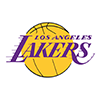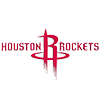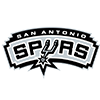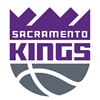DraftKings 2019 Western Conference Odds
+170
1.7 to 1Lakers
31.5% implied probability

+180
1.8 to 1Clippers
30.4% implied probability

+650
6.5 to 1Rockets
11.3% implied probability

+1000
10 to 1Jazz
7.7% implied probability

+1200
12 to 1Nuggets
6.5% implied probability

+1600
16 to 1Mavericks
5.0% implied probability

+5000
50 to 1Trail Blazers
1.7% implied probability

+8000
80 to 1Grizzlies
1.1% implied probability

+8000
80 to 1Thunder
1.1% implied probability

+8000
80 to 1Spurs
1.1% implied probability

+13000
130 to 1Pelicans
0.6% implied probability

+15000
150 to 1Timberwolves
0.6% implied probability

+15000
150 to 1Suns
0.6% implied probability

+15000
150 to 1Kings
0.6% implied probability

+25000
250 to 1Warriors
0.3% implied probability